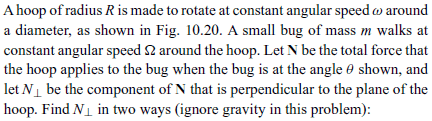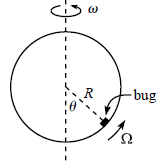# Components of a force in an inertial and a non-inertial reference frame

pablet
Homework Statement:
Find the perpendicular component of N in an inertial frame and in a non-inertial frame
Relevant Equations:
newton equation for non inertial frames
I know that the perpendicular force is the Coriolis force, but I don't know how to get it